# NCERT Solutions for Class 8 Maths Exercise 15.3## myCBSEguide App

CBSE, NCERT, JEE Main, NEET-UG, NDA, Exam Papers, Question Bank, NCERT Solutions, Exemplars, Revision Notes, Free Videos, MCQ Tests & more.

NCERT solutions for Class 8 Maths Introduction to Graphs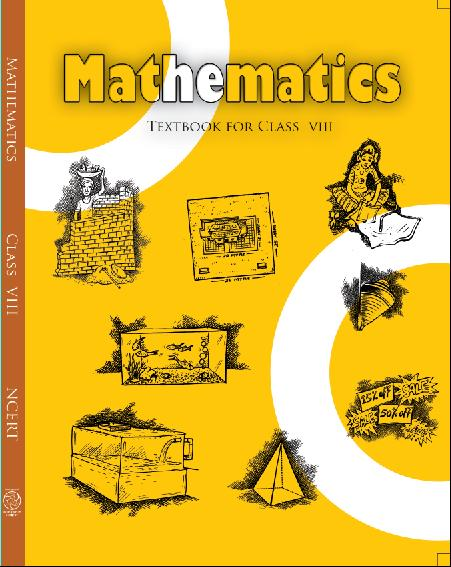## NCERT Solutions for Class 8 Maths Introduction to Graphs

Class –VIII Mathematics(Ex. 15.3)

NCERT SOLUTION

###### 1. Draw the graphs for the following tables of values, with suitable scales on the axes.

(a) Cost of apples

 No. of apples 1 2 3 4 5 Cost (in Rs.) 5 10 15 20 25

(b) Distance travelled by a car

 Time (in hours) 6 a.m. 7 a.m. 8 a.m. 9 a.m. Distance (in km) 40 80 120 160

(i)   How much distance did the car cover during the period 7.30 a.m. to 8 a.m?
(ii) What was the time when the car had covered a distance of 100 km since it’s start?

(c) Interest on deposits for a year.

 Deposit(inRs.) 1000 2000 3000 4000 5000 Simple Interest (inRs.) 80 160 240 320 400

(i)     Does the graph pass through the origin?
(ii)    Use the graph to find the interest on Rs 2500 for a year.
(iii)   To get an interest of Rs 280 per year, how much money should be deposited?

Ans.  (a)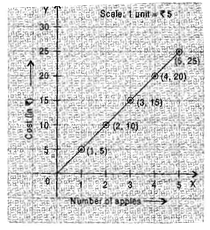(b) (i) The car covered 20 km distance.

(ii)It was 7.30 am, when it covered 100 km distance.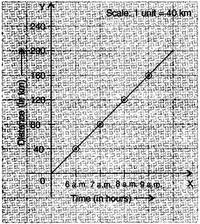(c) (i)   Yes, the graph passes through the origin.

(ii)  Interest on 2500 is 200 for a year.

(iii) 3500 should be deposited for interest of 280.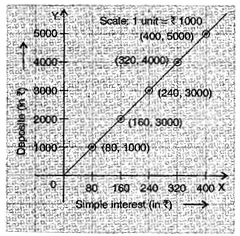NCERT Solutions for Class 8 Maths Exercise 15.3

###### 2. Draw a graph for the following.

(i)

 Side of Square(in cm) 2 3 3.5 5 6 Perimeter(in cm) 8 12 14 20 24

Is it a linear graph?

(ii)

 Side of Square(in cm) 2 3 4 5 6 Area (in cm2) 4 9 16 25 36

Is it a linear graph?

Ans. (i)  Yes, it is a linear graph.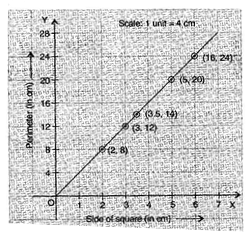(ii) No, it is not a linear graph because the graph does not provide a straight line.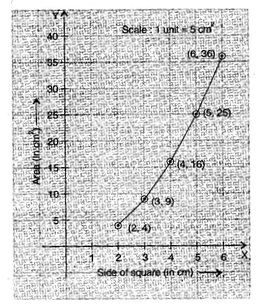## NCERT Solutions for Class 8 Maths Exercise 15.3

NCERT Solutions Class 8 Mathematics PDF (Download) Free from myCBSEguide app and myCBSEguide website. Ncert solution class 8 Mathematics includes text book solutions from Class 8 Maths Book . NCERT Solutions for CBSE Class 8 Maths have total 16 chapters. 8 Maths NCERT Solutions in PDF for free Download on our website. Ncert class 8 solutions PDF and Maths ncert class 8 PDF solutions with latest modifications and as per the latest CBSE syllabus are only available in myCBSEguide.

## CBSE app for Class 8

To download NCERT Solutions for class 8 Social Science, Computer Science, Home Science,Hindi ,English, Maths Science do check myCBSEguide app or website. myCBSEguide provides sample papers with solution, test papers for chapter-wise practice, NCERT solutions, NCERT Exemplar solutions, quick revision notes for ready reference, CBSE guess papers and CBSE important question papers. Sample Paper all are made available through the best app for CBSE students and myCBSEguide website.### 2 thoughts on “NCERT Solutions for Class 8 Maths Exercise 15.3”

1. Interasting
?

2. Superbbb love this web site